# Problems set on motion (Kinematics Assignment 2)

Paragraph type question
(A) A Man X drops a stone from the fifteen floor of the building .A Man Y ascending in an elevator at a  constant speed v=10 m/s passed the Fifteen floor just as the stone is released
Question 1
Find the position, velocity of the stone as seen by the Man X at time t=2 sec
a. (19m ,19m/s)
b. (19.6m,19.6m/s)
c. (10m,10m/s)
d (11m,12m/s)
Question 2
Find the position, velocity of the stone relative to Man Y at 3 sec
a. (39m, 70m/s)
b. (19m, 70m/s)
c. (70m, 39 m/s)
d. (14 m, 29m/s)
Question 3
Find the acceleration of the stone with respect to Man X and Y.
a. (9.8 m/s2, 9.8m/s2)
b. (9 m/s2, 10 m/s2)
c. (10 m/s2, 10m/s2)
d. None of these
(B) A particle moves such a way that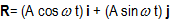where i and j are unit vectors along x and y direction. A and ω are constant
Question 4
Find the trajectory of the particle
1. Circle
2. Parabola
3. Eclipse
4. None of the above
Question 5
Find the average velocity of the particle from t=0 to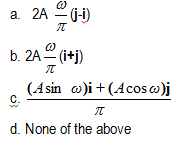Question 6
Find the scalar Product of Acceleration and velocity at any point t
a. 1
b. -1
c. 0
d. None of the above
Question 7
Find the tangential acceleration at any point of time t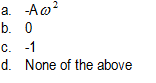Question 8
Find the radial acceleration at any point of time t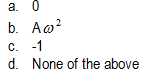Question 9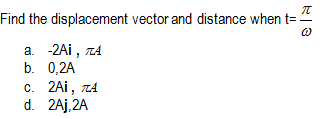(C) A gun is mounted on a train roof .Train is travelling with the velocity v in north direction. A car is also moving on a parallel track with train with velocity w in north direction. Two bullet are fired from the muzzle of the gun .Take north as positive and south as negative
Question 10
Bullet one is fired in the north direction with the muzzle velocity u. Find the velocity of the bullet as seen from the observer on the earth
a) u+v
b) u-v
c) u
d) v
Question 11
Find the velocity of the bullet as seen from the observer on the moving car
a u+v-w
b u-v-w
c u
d v
Question 12
Bullet one is fired in the south direction with the muzzle velocity u. Find the velocity of the bullet as seen from the observer on the earth
a u+v
b v-u
c u
d v
Question 13
Find the velocity of the second bullet as seen from the observer on the moving car
a u+v-w
b v-u-w
c u
d v
(D) A train is moving in the west direction with a velocity 15m/s.A monkey runs on the roof of the train against its motion with a velocity  5m/s with respect to train .Take the motion along west as positive
Question 14
Velocity of train relative to its driver
a. 0
b. 15 m/s
c. -15 m/s
d. 20 m/s
Question 15
What is the velocity of train with respect to monkey
a. 5m/s
b -5 m/s
c. 15 m/s
d -15 m/s
Question 16
Find the velocty of ground with respect to monkey
a. 5 m/s
b. -5 m/s
c. 10 m/s
d. -10 m/s
(E) Two particles start from the origin of the horizontal x-y plane. Particle A moves along +x axis with uniform velocity u. Particle B moves along -y axis with velocity u. i and j are the unit vectors along x and y direction
Question 17
Find the relative velocity of particle B wrt to A at time t
a -u(i+j)
b u(i+j)
c 0
d none of the above
Question 18
Find the relative velocity of particle A wrt to B at time t
a -u(i+j)
b u(i+j)
c 0
d none of the above
Question 19
find the relative position vector of particle A wrt to B at time t
a -ut(i+j)
b ut(i+j)
c 0
d none of the above
Answers
1.  (b)
2. (c)
3. (a)
4.  (a)
5. (a)
6. (c)
7. (b)
8. (b)
9. (a)
10.  (a)
11. (a)
12. (b)
13. (a)
14.  (a)
15. (a)
16. (d)
17.  (a)
18. (b)
19. (b)

Solutions

link to this page by copying the following text